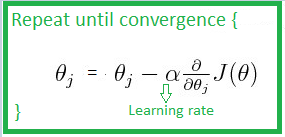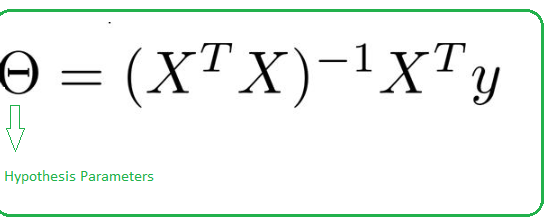# Difference between Gradient descent and Normal equation

Gradient descent is an optimization algorithm used to find the values of parameters of a function that minimizes a cost function. It is an iterative algorithm. We use gradient descent to update the parameters of the model. Parameters refer to coefficients in Linear Regression and weights in neural networks.Gradient descent can also converge even if the learning rate is kept fixed.

Normal Equation :
Normal Equation, an analytical approach used for optimization. It is an alternative for Gradient descent. Normal equation performs minimization without iteration.
Normal equations are equations obtained by setting equal to zero the partial derivatives of the sum of squared errors or cost function; normal equations allow one to estimate the parameters of multiple linear regression.Where
X = input features value
y = output value

If the termX is non-invertible or singular then we can use regularization.

Difference between Gradient Descent and Normal Equation.

1. In gradient descenet , we need to choose learning rate. In normal equation , no need to choose learning rate.
2. It is an iterative algorithm. It is analytical approach.
3. Gradient descent works well with large number of features. Normal equation works well with small number of features.
4. Feature scaling can be used. No need for feature scaling.
5. No need to handle non-invertibility case. If (X) is non-invertible , regularization can be used to handle this.
6. Algorithm complexity is O(k).
n is the number of features.
Algorithm complexity is O().
n is the number of features.
My Personal Notes arrow_drop_upCheck out this Author's contributed articles.

If you like GeeksforGeeks and would like to contribute, you can also write an article using contribute.geeksforgeeks.org or mail your article to contribute@geeksforgeeks.org. See your article appearing on the GeeksforGeeks main page and help other Geeks.

Please Improve this article if you find anything incorrect by clicking on the "Improve Article" button below.